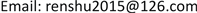﻿ 超几何分布高精度模型研究 Research on High-Precision Model of Hyper-Geometric Distribution

Vol.07 No.05(2018), Article ID:24940,3 pages
10.12677/AAM.2018.75060

Research on High-Precision Model of Hyper-Geometric Distribution

Renshu Piao

Institute of Xi’an Microelectronics and Technology, Xi’an ShaanxiReceived: Apr. 25th, 2018; accepted: May 11th, 2018; published: May 18th, 2018ABSTRACT

In order to derive the hyper-geometric distribution and multiplicative model, this paper classifies random variables according to their fractional properties. One is 0, and the other is 1, 2, ∙∙∙, n. Through the combined hyper-geometric branching model of two groups of elements, mathematical transformations were respectively performed, and a hyper-geometric distribution continuous multiplication model with no computer overflow was deduced. This model accurately calculates the probability value of all elements 0, 1, ∙∙∙, n of the hyper-geometric distribution, and the computer will not overflow when N is large.

Keywords:Hyper Geometric Distribution, Mathematical Transformation, Derivation, Random Variable, Branch, Model, Element1. 引言

2. 超几何分布模型建立

2.1. 随机变量元素d = 1, 2, ∙∙∙, n的超几何模型推导

${L}_{d1}=\frac{{C}_{D}^{{d}_{1}}{C}_{N-D}^{n-{d}_{1}}}{{C}_{N}^{n}}=\frac{\left(N-D\right)!}{N!}\cdot \frac{\left(N-n\right)!}{\left(N-D-n+{d}_{1}\right)!}\cdot \frac{n!}{\left(n-{d}_{1}\right)!}\cdot \frac{D!}{{d}_{1}!\left(D-{d}_{1}\right)!}\text{\hspace{0.17em}}\text{\hspace{0.17em}}\text{\hspace{0.17em}}\text{\hspace{0.17em}}{d}_{1}=1,2,\cdots ,n$ (1)

$\begin{array}{c}{L}_{d1}=\frac{\left(N-D\right)!}{\left(N-D\right)!\text{\hspace{0.17em}}\cdot \left(N-D+1\right)\cdot \left(N-D+2\right)\cdot \cdot \cdot N}\\ \text{\hspace{0.17em}}\text{\hspace{0.17em}}\cdot \frac{\left[\left(N-D\right)-\left(n-{d}_{1}\right)\right]!\text{\hspace{0.17em}}\cdot \left(N-D-n+{d}_{1}+1\right)\cdot \left(N-D-n+{d}_{1}+2\right)\cdot \cdot \cdot \left(N-n\right)}{\left[\left(N-D\right)-\left(n-{d}_{1}\right)\right]!}\\ \text{\hspace{0.17em}}\text{\hspace{0.17em}}\cdot \frac{\left(n-{d}_{1}\right)!\text{\hspace{0.17em}}\cdot \left(n-{d}_{1}+1\right)\cdot \left(n-{d}_{1}+2\right)\cdot \cdot \cdot n}{\left(n-{d}_{1}\right)!}\cdot \frac{\left(n-{d}_{1}\right)!\cdot \left(n-{d}_{1}+1\right)\cdot \left(n-{d}_{1}+2\right)\cdot \cdot \cdot n}{\left(n-{d}_{1}\right)!\text{\hspace{0.17em}}\cdot {d}_{1}!}\\ =\left(1-\frac{n-{d}_{1}}{N-D+1}\right)\cdot \left(1-\frac{n-{d}_{1}}{N-D+2}\right)\cdot \cdot \cdot \left(1-\frac{n-{d}_{1}}{N-D+D-{d}_{1}}\right)\cdot \left(1-\frac{N-n}{N-{d}_{1}+1}\right)\\ \text{\hspace{0.17em}}\text{\hspace{0.17em}}\cdot \left(1-\frac{N-n}{N-{d}_{1}+2}\right)\cdot \cdot \cdot \left(1-\frac{N-n}{N-{d}_{1}+{d}_{1}}\right)\cdot \left(1+\frac{D-{d}_{1}}{1}\right)\cdot \left(1+\frac{D-{d}_{1}}{2}\right)\cdot \cdot \cdot \left(1+\frac{D-{d}_{1}}{{d}_{1}}\right)\end{array}$

${L}_{d1}=\prod _{k=1}^{D-d}\left(1-\frac{n-d}{N-D+k}\right)\cdot \prod _{k=1}^{d}\left(1-\frac{N-n}{N-d+k}\right)\cdot \prod _{k=1}^{d}\left(1+\frac{D-d}{k}\right)\text{\hspace{0.17em}}\text{\hspace{0.17em}}\text{\hspace{0.17em}}\text{\hspace{0.17em}}d=1,2,3,\cdots ,n$ (2)

2.2. 特殊随机变量d = 0的超几何分布模型

${L}_{d2}=\frac{{C}_{N-D}^{n}}{{C}_{N}^{n}}=\frac{\left(N-D\right)}{N!}\cdot \frac{\left(N-n\right)!}{\left(N-D-n\right)!}$ (3)

${L}_{d2}=\prod _{k=1}^{D}\left(1-\frac{n}{N-D+k}\right)$ (4)

2.3. 超几何分布完整模型

${L}_{d}=\frac{{C}_{D}^{d}{C}_{N-D}^{n-d}}{{C}_{D}^{n}}=\left\{\begin{array}{l}\prod _{k=1}^{D-d}\left(1-\frac{n-d}{N-D+k}\right)\cdot \prod _{k=1}^{d}\left(1-\frac{N-n}{N-d+k}\right)\cdot \prod _{k=1}^{d}\left(1+\frac{D-d}{k}\right)\text{\hspace{0.17em}}\text{\hspace{0.17em}}\text{\hspace{0.17em}}\text{\hspace{0.17em}}\text{\hspace{0.17em}}d=1,2,\cdots ,n\\ \prod _{k=1}^{D}\left(1-\frac{n}{N-D+k}\right)\text{\hspace{0.17em}}\text{\hspace{0.17em}}\text{\hspace{0.17em}}\text{\hspace{0.17em}}\text{\hspace{0.17em}}\text{\hspace{0.17em}}\text{\hspace{0.17em}}\text{\hspace{0.17em}}\text{\hspace{0.17em}}\text{\hspace{0.17em}}\text{\hspace{0.17em}}\text{\hspace{0.17em}}\text{\hspace{0.17em}}\text{\hspace{0.17em}}\text{\hspace{0.17em}}\text{\hspace{0.17em}}\text{\hspace{0.17em}}\text{\hspace{0.17em}}\text{\hspace{0.17em}}\text{\hspace{0.17em}}\text{\hspace{0.17em}}\text{\hspace{0.17em}}\text{\hspace{0.17em}}\text{\hspace{0.17em}}\text{\hspace{0.17em}}\text{\hspace{0.17em}}\text{\hspace{0.17em}}\text{\hspace{0.17em}}\text{\hspace{0.17em}}\text{\hspace{0.17em}}\text{\hspace{0.17em}}\text{\hspace{0.17em}}\text{\hspace{0.17em}}\text{\hspace{0.17em}}\text{\hspace{0.17em}}\text{\hspace{0.17em}}\text{\hspace{0.17em}}\text{\hspace{0.17em}}\text{\hspace{0.17em}}\text{\hspace{0.17em}}\text{\hspace{0.17em}}\text{\hspace{0.17em}}\text{\hspace{0.17em}}\text{\hspace{0.17em}}\text{\hspace{0.17em}}\text{\hspace{0.17em}}\text{\hspace{0.17em}}\text{\hspace{0.17em}}\text{\hspace{0.17em}}\text{\hspace{0.17em}}\text{\hspace{0.17em}}\text{\hspace{0.17em}}\text{\hspace{0.17em}}\text{\hspace{0.17em}}\text{\hspace{0.17em}}\text{\hspace{0.17em}}d=0\end{array}$ (5)

3. 结束语

Research on High-Precision Model of Hyper-Geometric Distribution[J]. 应用数学进展, 2018, 07(05): 501-503. https://doi.org/10.12677/AAM.2018.75060

1. 1. 马彦恒, 韩九强, 等. 测试性评估与验证的超几何分布法[J]. 西安交通大学学报, 2009, 43(3): 42-45.

2. 2. 范晓冬, 孙蕾. 计数抽样检验方案批接收概率的计算方法[J]. 渤海大学学报, 2005, 26(2): 102-104.

3. 3. 杨玉梅, 李峰. 超几何分布概率任意精度算法及其实现[J]. 徐州师范大学学报(自然科学版), 2010, 28(2): 20-25.

4. 4. 仲崇新. 二项概率和超几何概率的近似计算及其误差[J]. 数学的实践与认识, 1991(1): 55-61.

5. 5. 王学民. 概率论与数理统计[M]. 上海: 复旦大学出版社, 2011.

6. 6. 肖明森. 关于超几何分布简化计算方法的探讨[J]. 数理统计与管理, 1988(4): 34-37.